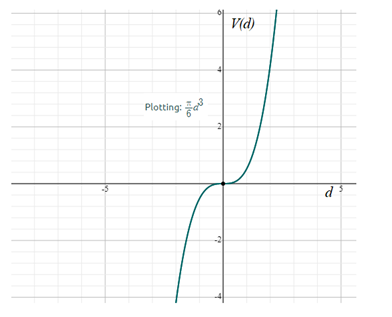# From the given statement, take the cube of the diameter mean d 3 then multiply by π and divide by 6 as: V ( d ) = d 3 π 6 ⇒ V ( d ) = π 6 d 3### Precalculus: Mathematics for Calcu...

6th Edition
Stewart + 5 others
Publisher: Cengage Learning
ISBN: 9780840068071### Precalculus: Mathematics for Calcu...

6th Edition
Stewart + 5 others
Publisher: Cengage Learning
ISBN: 9780840068071

#### Solutions

Chapter 2.1, Problem 68E
To determine

## From the given statement, take the cube of the diameter mean d3 then multiply by π and divide by 6 as:  V(d)=d3π6⇒V(d)=π6d3

Expert Solution

The required values of the given statement are shown in table as below:

dV(d)10.52352 4.18873   14.13710010.5235

### Explanation of Solution

Given information: Given statement is that, take the cube of the diameter mean d3 then multiply by π and divide by 6 as:

Calculation:

Take and Plug d=1 in V(d)=π6d3

V(1)=π6(1)3          =0.5235

Similarly,

V(2)=π6(2)3          =4.1887

V(3)=π6(3)3          =14.1371V(0)=π6(0)3        =0V(1)=π6(1)3        =0.5235

Thus, the required values of the given statement are shown in table as below:

d V( d ) 1 0.5235 2  4.1887 3    14.1371 0 0 1 0.5235

Graph of the function V(d)=π6d3 as shown below:### Have a homework question?

Subscribe to bartleby learn! Ask subject matter experts 30 homework questions each month. Plus, you’ll have access to millions of step-by-step textbook answers!